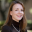# Standards-based Linear Algebra

This semester I’m teaching our introductory linear algebra course. As I did for Calculus II, I’ve implemented a standards-based assessment system. I’ve taken our course content and split it into “standards”, or little pieces of mathematics that I want my students to master. These standards are grouped together by what I call “Big Questions”. Here is what we’ve covered so far this semester:

• Big Question #1: What are the tools for solving systems of linear equations?
• 1.1: I can solve systems of linear equations using row operations. I can use Gaussian elimination with back-substitution to solve systems of linear equations. I can use Gauss-Jordan elimination to solve systems of linear equations.
• 1.2: I can characterize the solutions to systems of linear equations using appropriate notation and vocabulary.
• 1.3: I can use matrix inverses to solve systems of linear equations.
• 1.4: I can find and use an LU-factorization of a matrix to solve a system of linear equations.
• Big Question #2: What is the fundamental structure of the algebra of matrices?
• 2.1: I can perform algebraic operations with matrices, including addition, subtraction, scalar multiplication, and matrix multiplication. I can compute the transpose of matrices.
• 2.2: I can find the inverse of matrices using Gaussian elimination. I can find the inverse of matrices using a product of elementary matrices.
• 2.3: I can demonstrate theoretical connections about properties in the algebra of matrices.
• Big Question #3: How can we characterize invertible matrices?
• 3.1: I can find determinants using cofactor expansion. I can find determinants using row or column operations.
• 3.2: I can demonstrate theoretical connections between statements equivalent to “the matrix A is invertible.”
• 3.3: I can demonstrate theoretical connections between matrix equations, vector equations, and systems of linear equations, and their properties and solutions.
• Big Question #4: What are vector spaces & how can we describe them?
• 4.1: I can prove whether an algebraic structure is a vector space (or not) using the vector space axioms. I can prove whether or not a subset W of a vector space V forms a subspace. I can determine and characterize subspaces of \$\mathbb{R}^n\$.
• 4.2: I can write a proof showing whether a subset of vectors from a vector space forms a spanning set for the vector space (or not). I can write a proof to show whether a subset of vectors from a vector space is linearly independent (or not). I can determine whether a set of vectors forms a basis for a vector space. I can find the dimension of a vector space.
• 4.3: I can find a basis for the row space, the column space, or the null space of a matrix. I can determine the rank and nullity of a matrix. Given a consistent system Ax=b, I can describe the general solution in the form x=xp+xh
• 4.4: I can demonstrate knowledge of the theory of vector spaces by proving elementary results and theorems.

The remaining Big Questions are:

• Big Question #5: What are inner product spaces and how can we describe them?
• Big Question #6: What kinds of functions map one vector space into another while preserving vector space operations?
• Big Question #7: What are eigenvalues and why are they useful?

Our first exam was last week, so today has been Re-Assessment Central in my office. I’ll hand back our exams tomorrow and I’m hoping to talk with my students more about standards-based grading and how they can improve their standing in the course.

## 2 thoughts on “Standards-based Linear Algebra”

1.Andy Rundquist on said:

This is very interesting to me because I’m teaching a “math methods for physicists” course that’s also SBG. The linear algebra part for me would really only be what you’re calling 1, 3, and 7. As I’m prepping (and figuring out my “I can …” statements) I’m lamenting that I don’t have time for the others you mention here.

Question: Does it bother them that 5-7 aren’t as fleshed out?

•Kate Owens on said:

My totally honest answer is “I don’t know.” 🙂 But I am not sure they have even paid attention to questions 5-7 yet. I’ve been producing documentation on the fly, as we go. I have shown them all of the “Big Questions” listed above but not the bullet points underneath. Partly, this is because I’m still editing my wording. Partly, this is because I am not 100% sure what we will have time to get to. I’m teaching the same course again next semester & hopefully I’ll have everything ready to go in January, but right now I’m just building stuff on the fly.